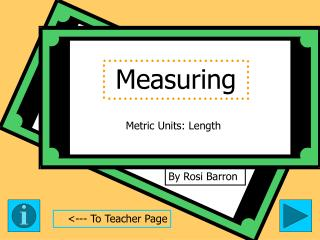DownloadDownload PresentationMeasuring

# Measuring

Télécharger la présentation## Measuring

- - - - - - - - - - - - - - - - - - - - - - - - - - - E N D - - - - - - - - - - - - - - - - - - - - - - - - - - -
##### Presentation Transcript

1. Measuring Metric Units: Length By Rosi Barron <--- To Teacher Page

2. Fifth Grade Measuring: Metric Length Measuring • Tips: -Teachers, please read through the whole presentation prior to the activity. -Each activity is meant to be used in addition to your classroom teaching. -Each activity includes a journal component. • Objectives: TSW determine the appropriate metric unit for measuring length. Teacher Page

3. These are the LengthMetric Units of Measurement. 1,000 millimeters (mm) = 1 meter (m) 100 centimeters (cm) = 1 meter 10 decimeters (dm) = 1 meter 1 kilometer (km) = 1,000 meters Journal each time you see this pad. Centimeter about as wide as your pinkie. Millimeter about as wide as a dime. Decimeter is equal to 10 centimeters.

4. Write A, B, or C and then tell why you chose this measurement as the most reasonable. Width of a window A. 10 cm B. 10 dm C. 10 km Diameter of a ring A. 15 mm B. 15 cm C. 15 km Length of a crayon A. 8 mm B. 8 cm C. 8 dm Length of a car A. 4 dm B. 4 m C. 4 km Click to see if you answered correctly --------->

5. Are these the choices you made? Width of a window A. 10 cm B. 10 dm C. 10 km Diameter of a ring A. 15 mm B. 15 cm C. 15 km Length of a crayon A. 8 mm B. 8 cm C. 8 dm Length of a car A. 4 dm B. 4 m C. 4 km Journal what else might have one of these measurements.